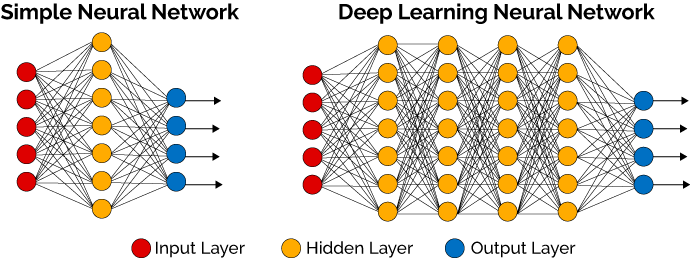Article

# Deep Learning 101

## A Light Introduction to Deep Learning

** Introduction **

In the context of Neural Networks and Machine Learning, what’s the first thing that comes to mind when you hear the term “Deep Learning”? If you follow your intuition, you probably thought of it as some mysterious form of learning that Neural Networks or other Machine Learning models undergo while trying to solve a problem. And that is mostly true! Let’s try to shed some light on this mystery in this article.

** What is Deep Learning? **

Deep Learning is a technique used in the world of Machine Learning, particularly involving Neural Networks, in which the input is progressively transformed into more abstract and higher-level representations. What does that mean? It means that with each step in the Deep Learning process, the input is understood better and better by the Neural Network, thereby taking us closer and closer to an approximate, yet accurate, answer.

That sounded a bit abstract so let’s understand the idea of Deep Learning by being more concrete. More specifically, let’s answer two core questions:

• Why does a Neural Network have layers?
• What does it mean for a Neural Network to learn deeply?

Why does a Neural Network have layers?

If we follow the historical trend of technology, we see that new technology eventually outdoes itself with significant improvements. Neural Networks, as awesome as they already were, underwent such changes to give rise to the concept of Deep Learning. Each layer in the neural network could be thought to represent a concept. By adding several such layers one after the other, the networks grows somewhat “deep”. This “deep” representation of the neural network’s “learning” is the core essence behind the naming of the term “Deep Learning”.

To be more specific, a Neural Network is said to be deep if it has more than one hidden layer. At this level of abstraction, the problem can be considered to be sufficiently complex enough to require Deep Learning in order to reap useful results. But even simple problems can undergo Deep Learning, which can also produce useful and surprising results.What does it mean for a Neural Network to learn deeply?

Image Recognition is a classic problem to understand how Deep Learning works. Although we humans are incredibly good at recognizing images and objects around us, we owe it to the magical processes in the brain and the overall human body that underlie this seemingly instant ability. For a machine, however, this doesn’t come so naturally. But with a Deep Neural Network (a Neural Network with relatively large number of layers), the results can sometimes even make a mockery of human ability!

For example, when we humans notice a book on the bookshelf, we immediately recognize it as a book because of the years of neural programming that has made us naturally recognize a book. However, there are many complex processes executing under the hood that recognize the shape and size of the object, whether or not it has an edge, and so on. Those unconscious processes finally produce a result that makes us think that we know for sure that the object is a book.

A similar process takes place in the case of Deep Learning. There are several layers of representations of the object that is being “deeply learned”. For example, let us consider a robot that is trying to identify whether an object is a book or not using Deep Learning. The robot first takes in the input through the first layer. At this point the object is just a collection of raw data for the robot. However, upon transforming this data in a special way, several patterns begin to emerge, such as the shape, size, number of edges, texture. Upon transforming these patterns, even more complex patterns begin to emerge, which upon several layers of transformation results in a high-level structure - a book!Can you think of other examples where machines can use Deep Learning to perform humanlike tasks? Can you apply a similar reasoning to digit recognition?

Conclusion

In this way, Deep Learning is a process that uncovers low-level patterns in the problem that is being solved and assembles them into high-level patterns through a series of layer-by-layer transformations. Such transformations are best accommodated by the use of a Neural Network. The terms Neural Network and Deep Learning go hand in hand - the former is a Machine Learning model that is used to solve problems in a humanlike fashion, whereas the latter is the mysterious mechanism by which these problems are solved. But we hope we’ve demystified the process to some extent in this article!